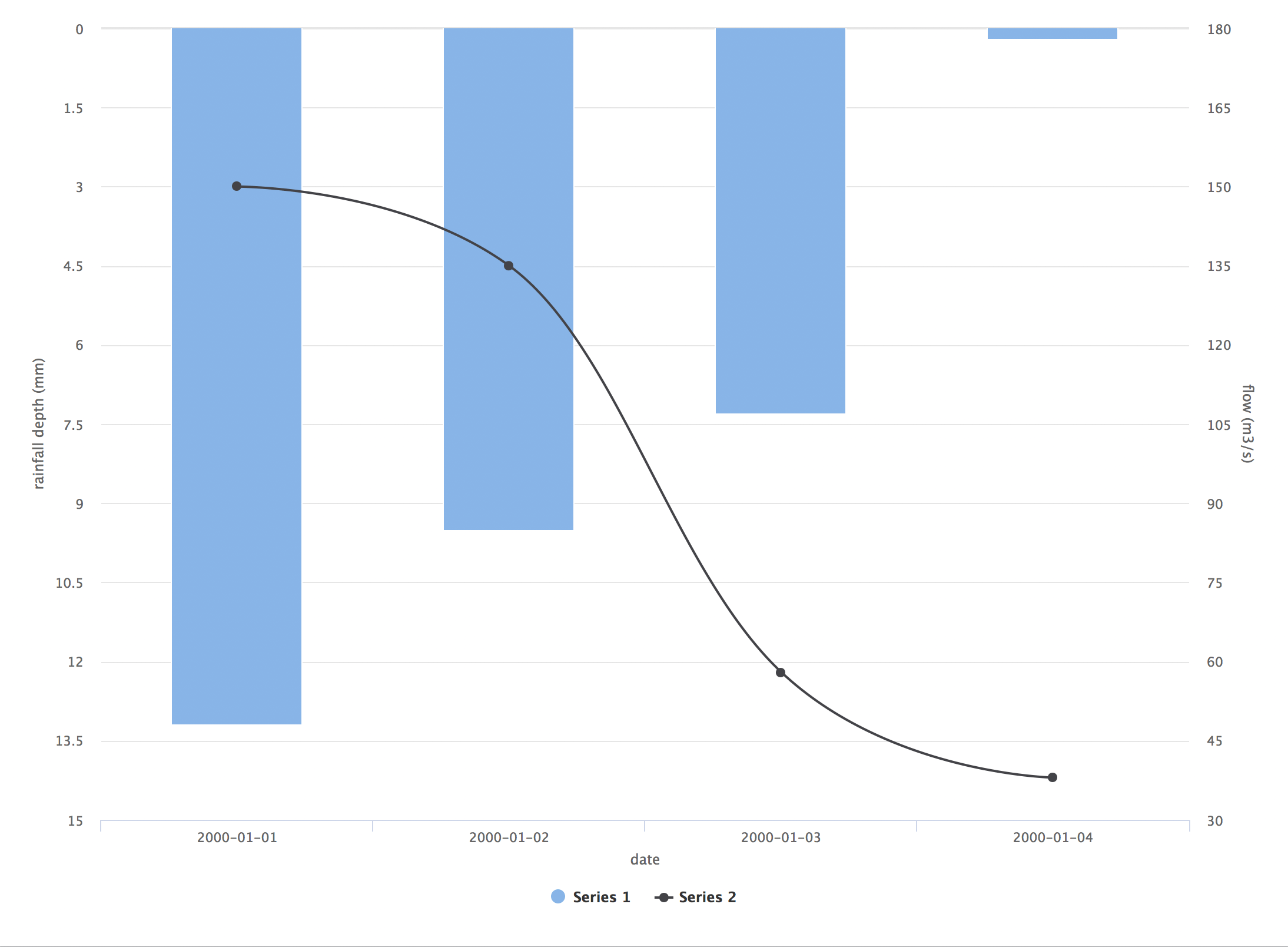[This article was first published on R – What You're Doing Is Rather Desperate, and kindly contributed to R-bloggers]. (You can report issue about the content on this page here)
Want to share your content on R-bloggers? click here if you have a blog, or here if you don't.

Dual y-axes: yes or no? What about if one of them is also reversed, i.e. values increase from the top of the chart to the bottom?

Judging by this StackOverflow question, hydrologists are fond of both of these things. It asks whether `ggplot2` can be used to generate a “rainfall hyetograph and streamflow hydrograph”, which looks like this:

My first thought was “why?” but perhaps, as suggested on Twitter, the chart signifies rain falling from above.

My view (and one held more widely) is that dual axes are to be discouraged unless (1) the variables measured in each case are directly comparable with regard to both magnitude and units, and (2) they don’t lead to clutter or visual confusion. This is why `ggplot2` makes it difficult to implement them, although there is limited support in recent version (see e.g. these examples).

I suggested using instead a javascript library, such as Highcharts. It’s a measure of how good the `highcharter` package is that having been using it for only a day, I was able to throw together an initial solution pretty quickly:

```library(highcharter)
library(dplyr)

rainfall <- data.frame(date = as.Date(rep(c("2000-01-01", "2000-01-02", "2000-01-03", "2000-01-04"), 2), "%Y-%m-%d"),
value = c(13.2, 9.5, 7.3, 0.2, 150, 135, 58, 38),
variable = c(rep("rain", 4), rep("discharge", 4)))

hc <- highchart() %>%
hc_yAxis_multiples(list(title = list(text = "rainfall depth (mm)"), reversed = TRUE),
list(title = list(text = "flow (m3/s)"),
opposite = TRUE)) %>%
hc_add_series(data = filter(rainfall, variable == "rain") %>% mutate(value = -value) %>% .\$value, type = "column") %>%
hc_add_series(data = filter(rainfall, variable == "discharge") %>% .\$value, type = "spline", yAxis = 1) %>%
hc_xAxis(categories = dataset\$rain.date, title = list(text = "date"))
hc
```

Here’s the interactive result. And here’s a screenshot:So it can be done and if that’s a “standard” plot in hydrology, I suppose it needs to be done…but given free rein, it’s not how I’d do it. Here’s a `ggplot2` alternative, using a common x-axis and facets for different variables.

```ggplot() +
facet_grid(variable ~ ., scales = "free", switch = "y", labeller = labeller(variable = c("rain" = "rainfall depth (mm)", "discharge" = "flow m3/s"))) +
geom_col(data = filter(rainfall, variable == "rain"), aes(date, value), fill = "skyblue3) +
theme_bw() +
geom_line(data = filter(rainfall, variable == "discharge"), aes(date, value)) +
labs(title = "A rainfall hyetograph and streamflow hydrograph")
```

Result:I’d argue that this approach still allows for visual comparison of the two variables, without implying that they measure the same thing.

Filed under: R, statistics Tagged: ggplot2, highcharter, highcharts, hydrology, package